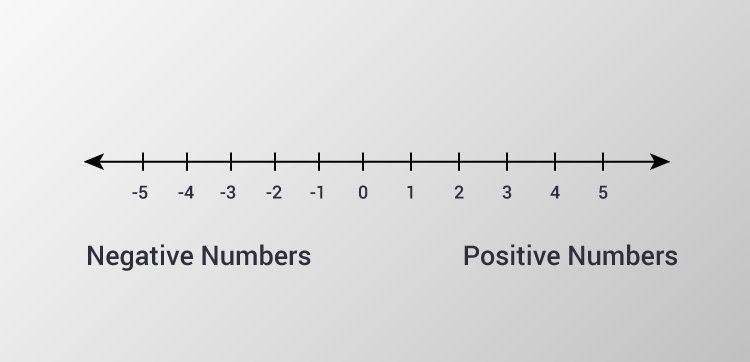# C Program to Check Whether a Number is Positive or Negative

In this example, you will learn to check whether a number (entered by the user) is negative or positive.To understand this example, you should have the knowledge of the following C programming topics:

This program takes a number from the user and checks whether that number is either `positive` or `negative` or `zero`.

## Check Positive or Negative Using if...else

``````#include <stdio.h>
int main() {
double num;
printf("Enter a number: ");
scanf("%lf", &num);
if (num <= 0.0) {
if (num == 0.0)
printf("You entered 0.");
else
printf("You entered a negative number.");
} else
printf("You entered a positive number.");
return 0;
}

``````

You can also solve this problem using nested `if else` statement.

## Check Positive or Negative Using Nested if...else

``````#include <stdio.h>
int main() {
double num;
printf("Enter a number: ");
scanf("%lf", &num);

if (num < 0.0)
printf("You entered a negative number.");
else if (num > 0.0)
printf("You entered a positive number.");
else
printf("You entered 0.");

return 0;
}
``````

Output 1

```Enter a number: 12.3
You entered a positive number.
```

Output 2

```Enter a number: 0
You entered 0.
```